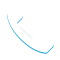### Statistical Analysis Using IBM SPSS Statistics (V25) – 0G51AG CR

Details

Course Code: 0G51AG

Brand: DS&BA – SPSS

Category: Analytics

Skill Level: Intermediate

Duration: 16.00H

Modality: CR

Audience

• Anyone who has worked with IBM SPSS Statistics and wants to become better versed in the basic statistical capabilities of IBM SPSS Statistics Base.
• Anyone who wants to refresh their knowledge and statistical experience.

Prerequisites

• Familiarity with basic concepts in statistics, such as measurement levels, mean, and standard deviation.
• Familiarity with the windows in IBM SPSS Statistics either by experience with IBM SPSS Statistics (version 18 or later) or completion of the IBM SPSS Statistics Essentials (V25) course.

Short Summary

In this course you will learn how to vizualize, describe, and test relationships between variables, using IBM SPSS Statistics (V25).

Overview

This course provides an application-oriented introduction to the statistical component of IBM SPSS Statistics. Students will review several statistical techniques and discuss situations in which they would use each technique, how to set up the analysis, as well as how to interpret the results. This includes a broad range of techniques for exploring and summarizing data, as well as investigating and testing relationships. Students will gain an understanding of when and why to use these various techniques as well as how to apply them with confidence, interpret their output, and graphically display the results.

Topic

Introduction to statistical analysis
• Identify the steps in the research process
• Principles of statistical analysis

Examine individual variables
• Identify measurement levels
• Chart individual variables
• Summarize individual variables
• Examine the normal distribution
• Examine standardized scores

Test hypotheses about individual variables
• Identify population parameters and sample statistics
• Examine the distribution of the sample mean
• Determine the sample size
• Test a hypothesis on the population mean
• Construct a confidence interval for the population mean
• Tests on a single variable: One-Sample T Test, Paired-Samples T Test, and Binomial Test

Test the relationship between categorical variables
• Chart the relationship between two categorical variables
• Describe the relationship: Compare percentages in Crosstabs
• Test the relationship: The Chi-Square test in Crosstabs
• Assumptions of the Chi-Square test
• Pairwise compare column proportions
• Measure the strength of the association

Test on the difference between two group means
• Compare the Independent-Samples T Test to the Paired-Samples T Test
• Chart the relationship between the group variable and scale variable
• Describe the relationship: Compare group means
• Test on the difference between two group means: Independent-Samples T Test
• Assumptions of the Independent-Samples T Test

Test on differences between more than two group means
• Describe the relationship: Compare group means
• Test the hypothesis of equal group means: One-Way ANOVA
• Assumptions of One-Way ANOVA
• Identify differences between group means: Post-hoc tests

Test the relationship between scale variables
• Chart the relationship between two scale variables
• Describe the relationship: Correlation
• Test on the correlation
• Assumptions for testing on the correlation
• Treatment of missing values

Predict a scale variable: Regression
• What is linear regression?
• Explain unstandardized and standardized coefficients
• Assess the fit of the model: R Square
• Examine residuals
• Include 0-1 independent variables
• Include categorical independent variables

Introduction to Bayesian statistics
• Bayesian statistics versus classical test theory
• Explain the Bayesian approach
• Evaluate a null hypothesis: Bayes Factor
• Bayesian procedures in IBM SPSS Statistics

Overview of multivariate procedures
• Overview of supervised models
• Overview of models to create natural groupings

Objectives

• Introduction to statistical analysis
• Examine individual variables
• Test hypotheses about individual variables
• Test the relationship between categorical variables
• Test on the difference between two group means
• Test on differences between more than two group means
• Test the relationship between scale variables
• Predict a scale variable: Regression
• Introduction to Bayesian statistics
• Overview of multivariate procedures
Tags :Call Now +27 72 266 2599